Latest Banking jobs   »

# Quantitative Aptitude Quiz For NABARD Grade A 2022- 8th August

Directions (1-5): In the following questions, two equations (I) and (II) are given. You have to solve both the equations and mark the appropriate answer.
(a) x<y
(b) x>y
(c) x≤y
(d) x≥y
(e) x=y or no relation.

Q1. I. (x+16)²=441
II. (y+22)²=961

Q2. I. x²-15x+56=0
II. y²-12y+35=0

Q3. I. x³=5832
II. y³=4096

Q4. I. (x-2) ²=5x-16
II. (y-4)²=9y-56

Q5. I. 42x²-71x+30=0
II. 6y²-17y+12=0

Directions (6-10): In each of these questions, two equations (I) and (II) are given. You have to solve both the equations and give answer
(a) if x>y
(b) if x≥y
(c) if x<y
(d) if x ≤y
(e) if x = y or no relation can be established between x and y.

Q6. I. 5x² + 28x + 15 = 0
II. 3y² – 7y – 6 = 0

Q7. I. x² – 14x – 15 = 0
II. y² – 19y + 60 = 0

Q8. I. 3x + 4y = 25
II. 4x + 3y = 24

Q9. I. x² = 64
II. y² – 18y + 80 = 0

Q10. I. 7x² + 20x = –12
II. y² + 9y = –18

Directions (11-15): In each of these questions, two equations (i) and (ii) are given. You have to solve both the equations and give answer
(a) if x>y
(b) if x≥y
(c) if x<y
(d) if x ≤y
(e) if x = y or no relation can be established between x and y.

Q11. (i) 4x² + 14x – 18 = 0
(ii) y² + 12y + 35 = 0

Q12. (i) 4x² + 17x – 42 = 0
(ii) y² + 21y + 90 = 0

Q13. (i) x² – 24x + 128 = 0
(ii) y² – 34y + 288 = 0

Q14. (i) 8x² – 30x + 28 = 0
(ii) 5y² – 8y + 3 = 0

Q15. (i). 9x²-15x+6=0
(ii). 12y²+20y–8=0

Solutions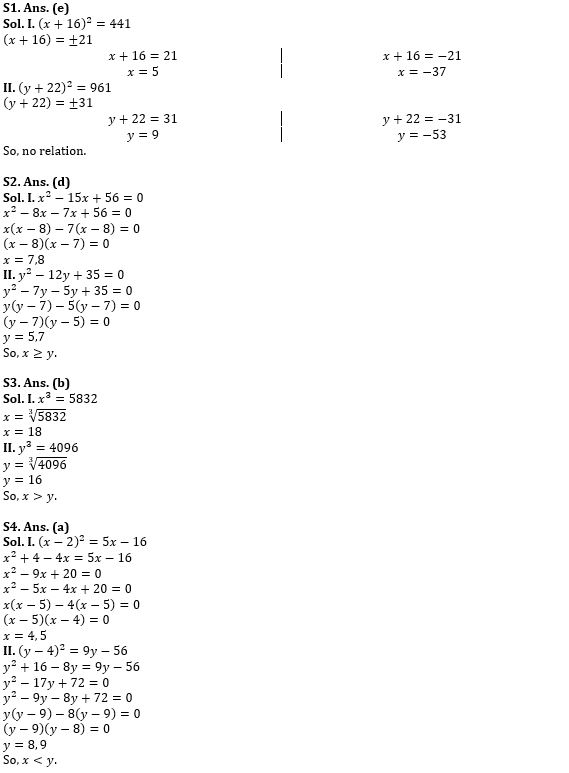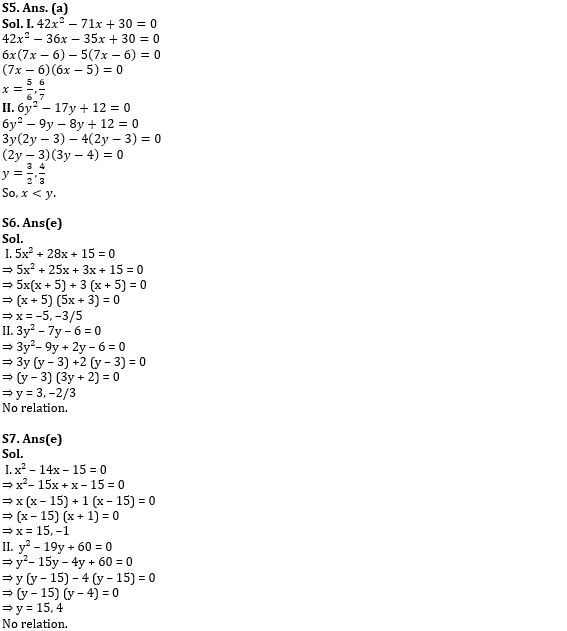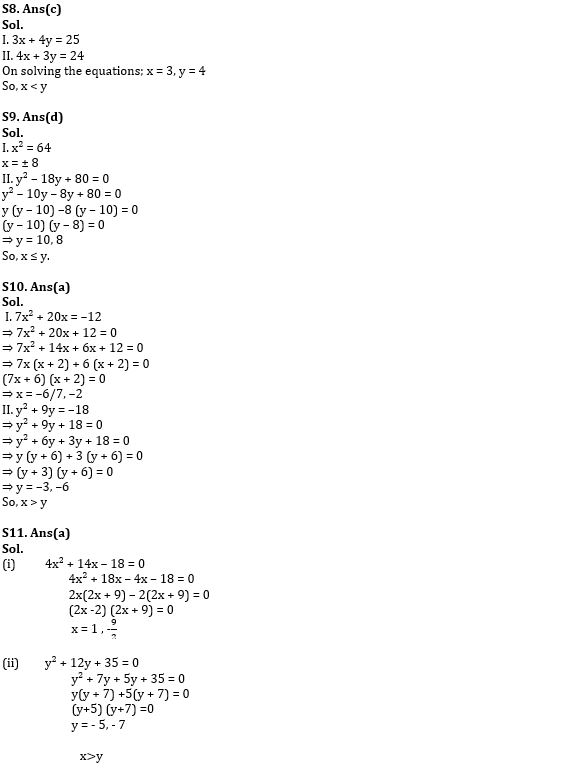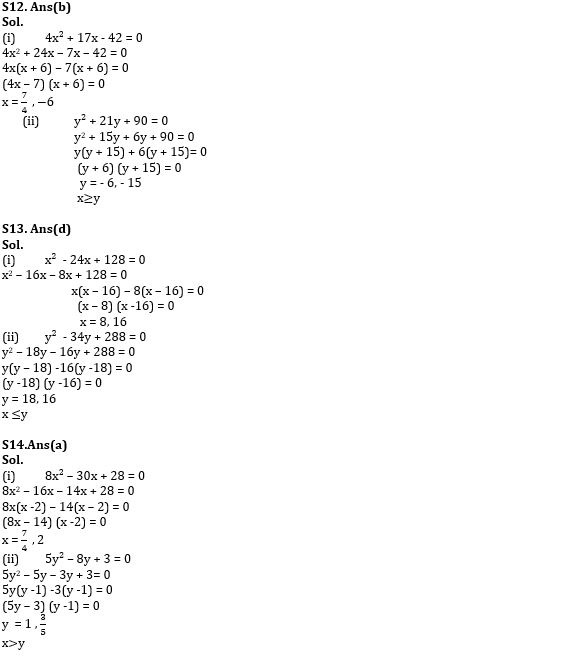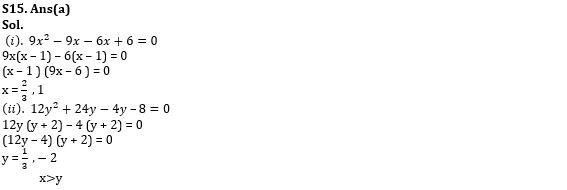#### Congratulations!Download Hindu Review of October 2021: Free PDFIncorrect details? Fill the form again here

Download Hindu Review of October 2021: Free PDF

Thank You, Your details have been submitted we will get back to you.
•American Express ties up with Nasscom to...
•Active credit cards drop 2.3 million in ...
•International Day For Older Persons 2022...
•Global Innovation Index Ranking 2022: In...
•International Translation Day 2022, Them...
•Debt marketplace Yubi partners with SBI ...